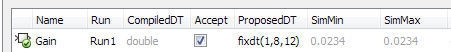### Fraction Length Greater Than Word Length

A fraction length greater than the word length of a fixed-point number occurs when the number has an absolute value less than one and contains leading zeros.

In the following example, the fixed-point tool proposed a data type with a fraction length that is four greater than the word length. A binary representation of this number consists of the binary point, four implied leading zeros, followed by the binary representation of the stored integer: . X X X X 0 1 1 0 0 0 0 0, where the x’s represent the implied zeros.### Negative Fraction Length

A negative fraction length occurs when the number contains trailing zeros before the decimal point.

In the following example, the fixed-point tool proposed a data type with a negative fraction length. A binary representation of this number consists of the binary representation of the stored integer, followed by seven implied zeros, and then the binary point: 0 1 1 1 1 1 0 1 X X X X X X X ., where the x’s represent the implied zeros.## Related Topics

##### SupportGet trial now